Balbharti Maharashtra State Board Class 6 Science Solutions Chapter 9 Motion and Types of Motion Notes, Textbook Exercise Important Questions and Answers.

## Maharashtra State Board Class 6 Science Solutions Chapter 9 Motion and Types of Motion

Class 6 Science Chapter 9 Motion and Types of Motion Textbook Questions and Answers

1. Identify the types of motion.

Question a.
Movement of the earth around the sun: …………… .
periodic, drcular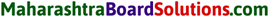Question b.
Movement of ceiling fan: …………… .
circular

Question c.
A rocket launched from the ground: ……….. .
linear

Question d.
A fish swimming in water: ……… .
random

Question e.
The plucked string of a sitar:………….. .
oscillatory motion2. Fill in the blanks.
(linear, non-linear, uniform linear, non-uniform linear, uniform circular, random, circular, non-uniform circular)

Question a.
If a ball is released from the terrace of a building, it comes down in ………… motion. On the other hand, it reaches the ground in ………… motion if it is thrown with a force away from the terrace in a direction parallel to the terrace.
uniform linear, non-uniform linear

Question b.
The motion of an aeroplane on the runway before take off is …………. .
linear

Question c.
The kite looking for its prey flies with …………. motion in the sky.
circularQuestion d.
Children sitting in a rotating giant wheel have ………. motion, while those sitting in a merry-go-round have a ………. motion.
uniform circular, non-uniform circular

3. How are we different?.

Question a.
Oscillatory motion and Linear motion.

 Oscillatory motion Linear motion 1. In oscillatory motion, body swings back and forth. e.g. Motion of a swing, motion of pendulum of a clock. 1. In linear motion, an object shows displacement in a straight line, e.g. A train in motion, motion of marching soldiers.

Question b.
Linear motion and Random motion

 Linear motion Random motion 1. Motion in a straight line is called linear motion. e.g. Motion of a train 1. The motion that changes its direction and speed continuously is called random motion, e.g. Motion of a bird.Question c.
Random motion and Oscillatory motion

 Random motion Oscillatory motion 1 The motion that changes its direction and speed continuously is called random motion, e.g. Motion of a butterfly 1. The motion of a body that is swinging back and forth is called oscillatory motion, e.g. Pendulum of a clock, the wing of a bird.

4. Explain in your own words, giving one example each.

Question a.
Linear motion
An object that shows displacement along a straight line is called linear motion, e.g. A vehicle moving on a road.Question b.
Oscillatory motion
The motion of a body swinging back and forth is called oscillatory motion, e.g. Motion of a pendulum of a clock.

Question c.
Circular motion
The motion of an object along a circular path is called circular motion, e.g. Motion of a ceiling fan.

Question d.
Random motion
The motion that changes its direction and speed continuously is called random motion, e.g. Motion of a butterfly.

Question e.
Periodic motion
The repetitive motion in which the moving object passes through a certain point again and again after a fixed period is called as periodic motion, e.g. The minute hand of a clock.Question a.
Which types of motion are seen in birds flying in the sky?

1. The birds flying in the sky exhibit random motion.
2. The wings of the birds show oscillatory motion.

Question b.
Write in detail about your experience of various types of motion while riding a bicycle on a road.

1. The cycle itself shows linear motion.
2. The wheels of the cycle show circular motion.
3. The cycle chain shows periodic motion, if the speed is uniform.
4. The handle bar shows random motion.6. Complete the puzzle using words for types of motion:

Question a.
Complete the puzzle using the words for types of motion.
1. A spring is stretched and one end is released.
2. A minute hand.
3. A see-saw.
4-5.  Children in a march past.
6. A stone rolling down a hillside.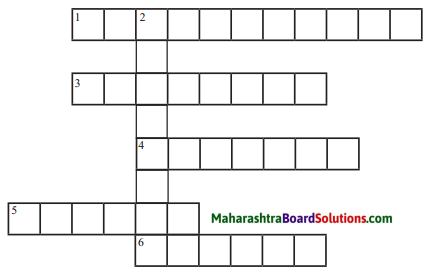1. Oscillatory
2. Circular
3. Periodic
4. Uniform
5. Linear
6. Random

Activity:

Question 1.
Make a list of various moving objects in the environment, and discuss the types of motion seen in them.

Class 6 Science Chapter 9 Motion and Types of Motion Important Questions and Answers

Identify the types of motion.

Question 1.
The movement of a see-saw.
oscillatory motion

Question 2.
The motion of a moving ant.
randomQuestion 3.
The marching army soldiers.
linear

Question 4.
A train approaching a station.
non-uniform linear

Question 5.
A meteor falling from the sky.
linear

Fill in the blanks with suitable words from those given in the bracket:
(linear, non-linear, uniform linear, non-uniform linear, uniform circular, random, circular, non-uniform circular)

Question 1.
The motion that changes its speed and direction continuously is called ………. .
randomChoose the correct alternative:

Question 1.
A baby is crawling. The motion is said to be …………….. .
(a) linear
(b) periodic
(c) circular
(d) random
(d) random

Question 2.
The children are having a 50m running race. The motion exhibited is ………….. motion.
(a) linear
(b) periodic
(c) random
(d) circular
(a) linearQuestion 3.
The motions of the hands of a clock are ………….. and …………. .
(a) periodic, linear
(b) periodic, circular
(c) non-linear, non-uniform
(d) circular, non-uniform
(b) periodic, circular

Question 4.
The motion of a pendulum of a clock is ……………. .
(a) linear
(b) oscillatory
(c) circular
(d) random
(b) oscillatory

Question 5.
The motion of a butterfly from one flower to another flower is an example of …………… motion.
(a) circular
(b) periodic
(c) linear
(d) random
(d) randomQuestion 6.
The distance traversed by an object in a unit time is called ……….. of that object.
(a) length
(b) motion
(c) speed
(d) displacement
(c) speed

Explain it in your own words, giving one example of each.

Question 1.
Uniform linear motion
When the distance traversed by an object along a straight line in unit time is the same, the motion is called as uniform linear motion, e.g. Motion of soldiers on parade.

Question 2.
Non-uniform linear motion
When the distance traversed by an object along a straight line in unit time keeps on changing, the motion is called non-uniform linear motion.
e.g. A girl coming down a slide.Question 3.
Non-linear motion
The motion of an object that does not move in a straight line is called non-linear motion.
e.g. Motion of a swing,

Question 4.
Speed
The distance traversed by an object in unit time is called the speed of that object.
e.g. A boy riding on a bicycle covers a distance of 15 kilometres in 3 hours.
Hence Speed = $$\frac{15}{3}$$ = 5 kilometres/hour

Question 1.
In which muscial instruments can you see oscillatory motion?
Vibrating diaphragm of tabla, drum, dhol and strings of sitar, guitar show oscillatory motions.Question 2.
With reference to types of motion, complete the table below.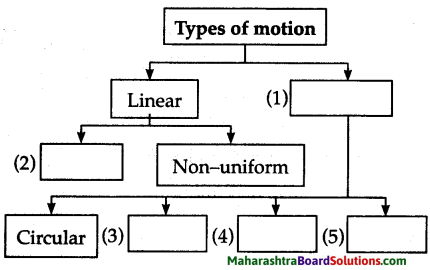1. Non-linear
2. Uniform
3. Oscillatory
4. Periodic
5. Random.

Rita and Geetha are friends travelling from Mumbai to Pune. Seetha had come to the station to see her friends off. After 15 minutes train starts moving. Rita and Geetha feel that Seetha and the vendors on platform are moving backwards. Whereas, Seetha feels that Rita and Geetha are moving forward. Rita feels Geetha is not in motion and Geetha also feels Rita is not in motion. Can you say why?

Question a.
Are Rita and Geetha in motion?
Rita and Geetha are not in motion as far as each other are concerned, as no displacement takes place. Both are in a train. For Seetha, both of them are in motion.Question b.
Are the vendors and Seetha in motion?
No, the vendors and Seetha are not in motion. But Rita and Geetha feel as if they are moving backwards.

Question c.
What can you conclude from this passage?
An object which is in motion for one person may not be in motion for another. This shows that motion is relative.

Observe and discuss:

Question 1.
Observe the figure and classify the type of motion.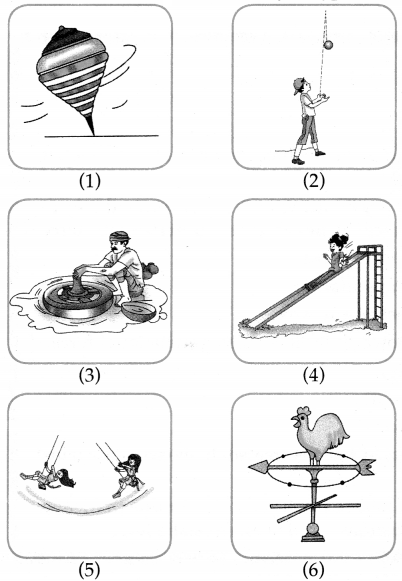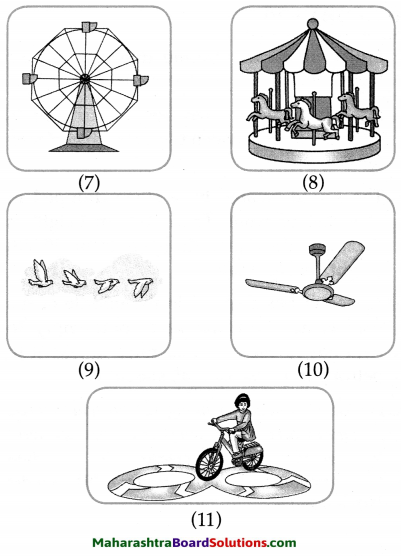1. Circular motion
2. Linear motion
3. Circular motion
4. Linear motion
5. Oscillatory motion
6. Oscillatory motion
7. Circular motion
8. Circular motion
9. Bird – Random; Wings – Oscillatory motion
10. Circular motion
11. Linear, circularCan you tell?

Question 1.
While chasing a butterfly in a garden, do you run along a definite path or in the same direction all the time?